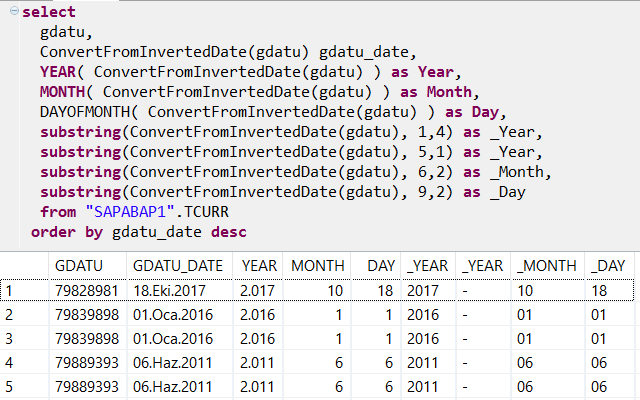SAP ABAP Programming and HANA Database Tutorials Development resources, articles, tutorials, code samples and tools and downloads for ASP.Net, SQL Server, R Script, Windows, Windows Phone, AWS, SAP HANA and ABAP, like SAP UI5, Screen Personas, etc.
 Home Articles News IT Jobs Tools Sample Chapters Trainers Blogs Forums Photos Files

Install SAP Free

# Convert Inverted Date in SAP HANA Database using SQL

In this SAP HANA SQLScript tutorial, SQL developers find tips to convert inverted date to date using SQL functions, from DATUM_INV domain to DATUM domain data type. In SAP, some date information isnot stored in data columns of type DATS, the expected preferred data type for date values. Instead, like the case in ABAP table TCURR Exchange Rates, date is stored in inverted date as for GDATU table field.

ABAP programmers developing SQL codes on SAP HANA Studio on SAP HANA database, who needs to convert inverted date column values of Exchange Rates table TCURR, GDATU field can refer to ABAP conversion exit routine CONVERSION_EXIT_INVDT_OUTPUT.

``` HILF1 = '99999999' - INPUT. TRANSLATE HILF1(5) USING ' 0'. CONDENSE HILF1 NO-GAPS. OUTDATUM = HILF1. WRITE OUTDATUM TO OUTPUT DD/MM/YYYY. ```

As ABAP developers can understand easily, the inverted date is simply calculated by substracting the input date field from static '99999999' value.
This conversion between DATE and Inverted Date parameters is something SQL developers can easily handle on SAP HANA database

For example, to see the corresponding date information on TCURR currency rates table GDATU column, following SQL Select can be executed.

``` select  gdatu,  to_date( to_nvarchar(99999999 - gdatu) ) gdatu_date,  YEAR( to_date( to_nvarchar(99999999 - gdatu) )) as Year,  MONTH( to_date( to_nvarchar(99999999 - gdatu) )) as Month,  DAYOFMONTH( to_date( to_nvarchar(99999999 - gdatu) )) as Day,  substring(to_date( to_nvarchar(99999999 - gdatu) ), 1,4) as _Year,  substring(to_date( to_nvarchar(99999999 - gdatu) ), 6,2) as _Month,  substring(to_date( to_nvarchar(99999999 - gdatu) ), 9,2) as _Day from "SAPS4S".TCURR order by gdatu ```

Here is the outputIf you check the above SQLScript code block the conversion from inverted date to date value is done by "to_date( to_nvarchar(99999999 - gdatu) )"

I also added additional fields in the SELECT list to show how SQL programmers can manipulate date data using HANA SQLScript Date functions and SubString() function to fetch related date parts as an alternative method.

The converted date in its string representation is "YYYY-MM-DD"

## SAP HANA SQLScript Function to Convert Inverted Date

Following user-defined function can be created on HANA database to use for inverted date conversion to DATE data type

``` CREATE FUNCTION ConvertFromInvertedDate (  invertedDate VARCHAR(8) ) returns toDate date LANGUAGE SQLSCRIPT SQL SECURITY INVOKER AS BEGIN  toDate := to_date( to_nvarchar(99999999 - :invertedDate) ); END ```

Here is how ConvertFromInvertedDate conversion function can be used in SQL codes

``` select  gdatu,  ConvertFromInvertedDate(gdatu) gdatu_date,  YEAR( ConvertFromInvertedDate(gdatu) ) as Year,  MONTH( ConvertFromInvertedDate(gdatu) ) as Month,  DAYOFMONTH( ConvertFromInvertedDate(gdatu) ) as Day,  substring(ConvertFromInvertedDate(gdatu), 1,4) as _Year,  substring(ConvertFromInvertedDate(gdatu), 5,1) as _Year,  substring(ConvertFromInvertedDate(gdatu), 6,2) as _Month,  substring(ConvertFromInvertedDate(gdatu), 9,2) as _Day  from "SAPABAP1".TCURR order by gdatu_date desc; ```

Output of the above SQL Select statement on a very seldomly used SAP system executed on TCURR field produced below data.SAP Tutorials

SAP Tutorial

SAP Forums

SAP Tools

SAP Transaction Codes Table

Meetup Sunumu 1